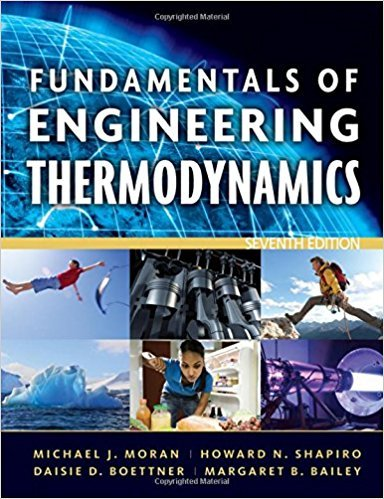×
×

# Solved: Otto, Diesel, and Dual Cycles Consider anISBN: 9780470495902 50

## Solution for problem 24P Chapter 9

Fundamentals of Engineering Thermodynamics | 7th Edition

• Textbook Solutions
• 2901 Step-by-step solutions solved by professors and subject experts
• Get 24/7 help from StudySoup virtual teaching assistantsFundamentals of Engineering Thermodynamics | 7th Edition

4 5 0 269 Reviews
30
5
Problem 24P

Otto, Diesel, and Dual Cycles Consider an air-standard Diesel cycle. Operating data at principal states in the cycle are given in the table below. The states are numbered as in Fig. 9.5. Determine

(a) the cutoff ratio.

(b) the heat addition per unit mass, in kJ/kg.

(c) the net work per unit mass, in kJ/kg.

(d) the thermal efficiency.

 State T (K) p (kPa) u (kJ/kg) h (kJ/kg) 1 380 100 271.69 380.77 2 1096.6 5197.6 842.40 1157.18 3 1864.2 5197.6 1548.47 2082.96 4 875.2 230.1 654.02 905.26Fig. 9.5 p–V and T–s diagrams of the air-standard Diesel cycle

Step-by-Step Solution:
Step 1 of 3

CHAPTER 15 RECONSTRUCTION THE WAR’S AFTERMATH IN THE SOUTH 1. Throughout the South, people were emotionally exhausted. a. Lives were shattered. 2. Property values collapsed. 3. Many large southern cities were in ruines. a. Richmond, Atlanta, and Columbia. 4. Emancipation wiped out \$4 billion invested in slavery. THE BATTLE OVER...

Step 2 of 3

Step 3 of 3

##### ISBN: 9780470495902

Since the solution to 24P from 9 chapter was answered, more than 300 students have viewed the full step-by-step answer. The answer to “Otto, Diesel, and Dual Cycles Consider an air-standard Diesel cycle. Operating data at principal states in the cycle are given in the table below. The states are numbered as in Fig. 9.5. Determine(a) the cutoff ratio.________________(b) the heat addition per unit mass, in kJ/kg.________________(c) the net work per unit mass, in kJ/kg.________________(d) the thermal efficiency.StateT (K)p (kPa)u (kJ/kg)h (kJ/kg)1380100271.69380.7721096.65197.6842.401157.1831864.25197.61548.472082.964875.2230.1654.02905.26 Fig. 9.5 p–V and T–s diagrams of the air-standard Diesel cycle” is broken down into a number of easy to follow steps, and 70 words. This full solution covers the following key subjects: Diesel, cycle, mass, standard, States. This expansive textbook survival guide covers 14 chapters, and 1501 solutions. This textbook survival guide was created for the textbook: Fundamentals of Engineering Thermodynamics, edition: 7. The full step-by-step solution to problem: 24P from chapter: 9 was answered by , our top Engineering and Tech solution expert on 07/20/17, 09:01AM. Fundamentals of Engineering Thermodynamics was written by and is associated to the ISBN: 9780470495902.

Unlock Textbook Solution